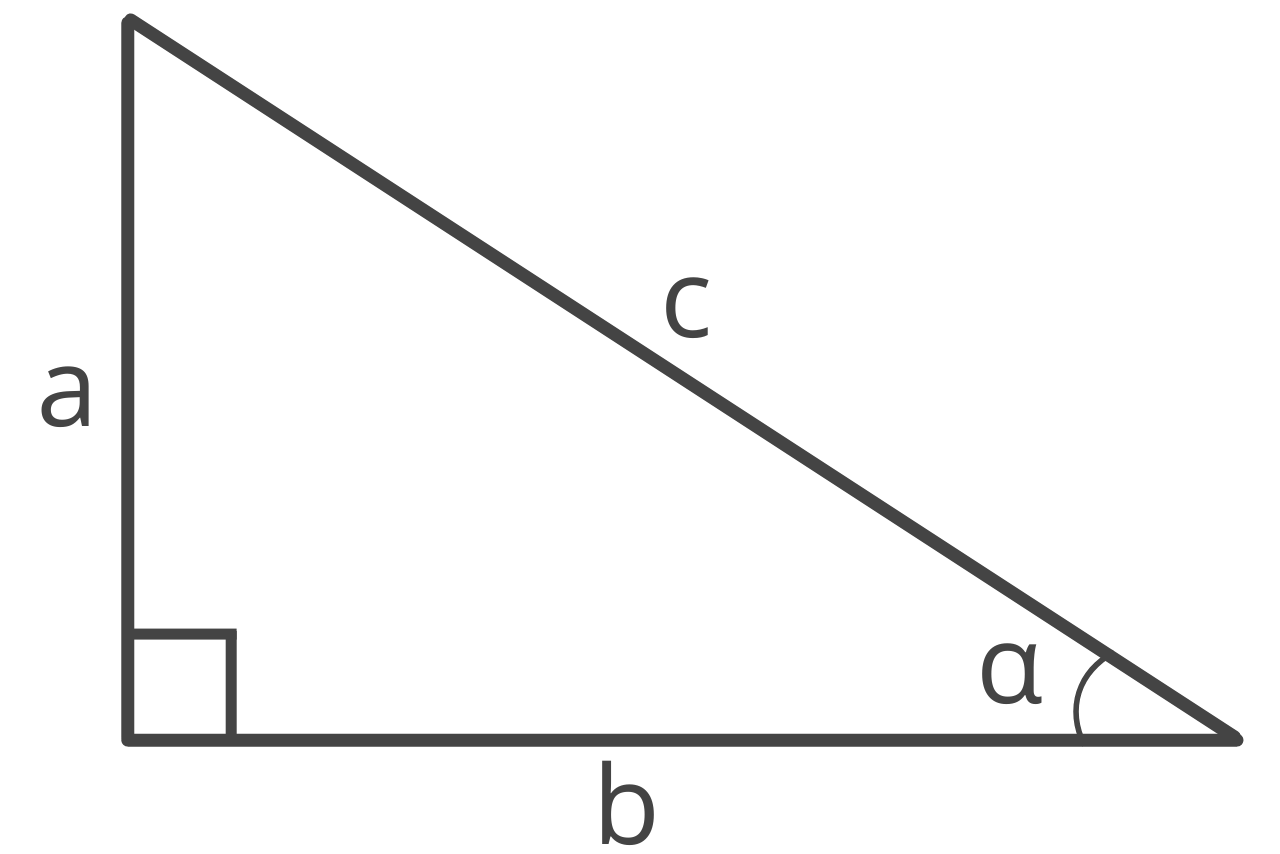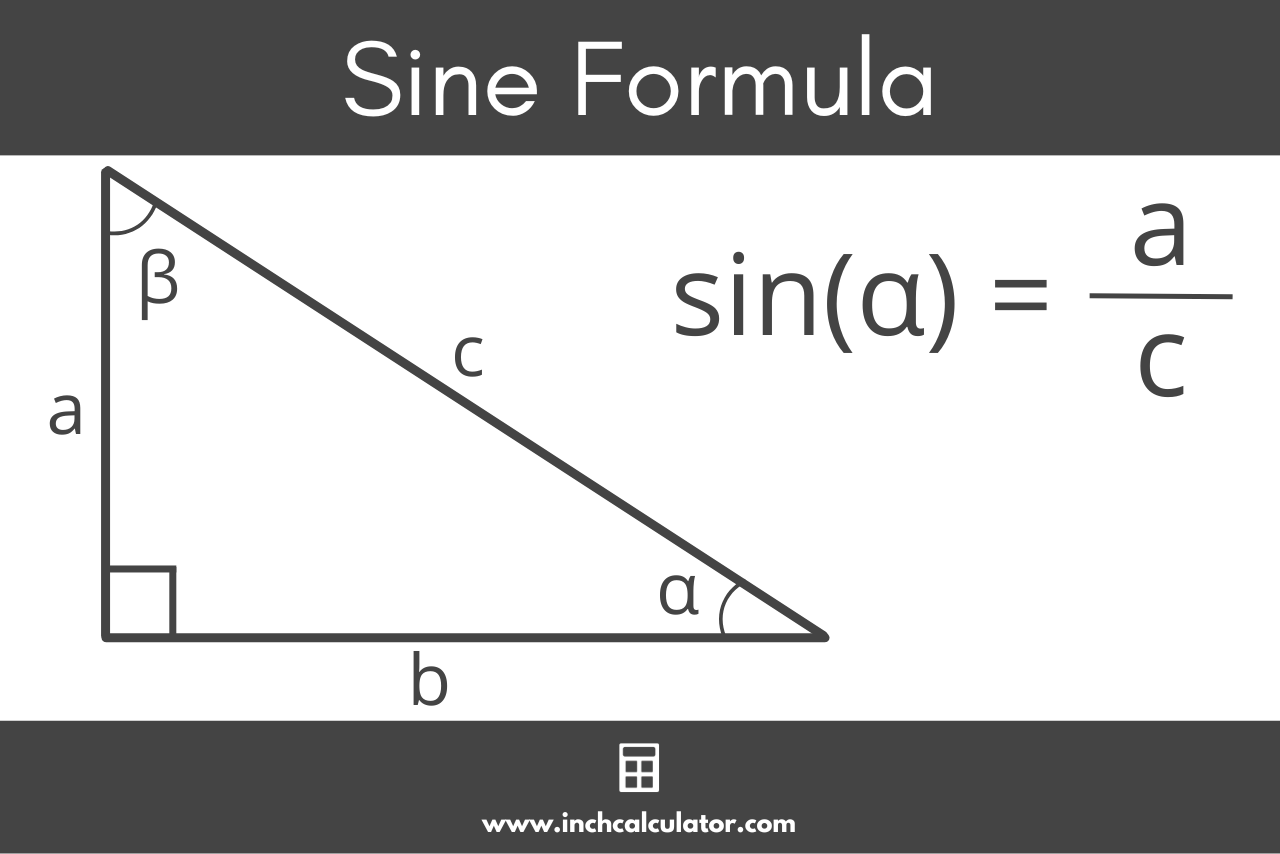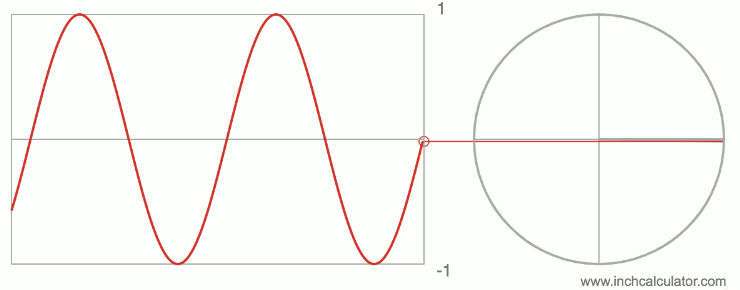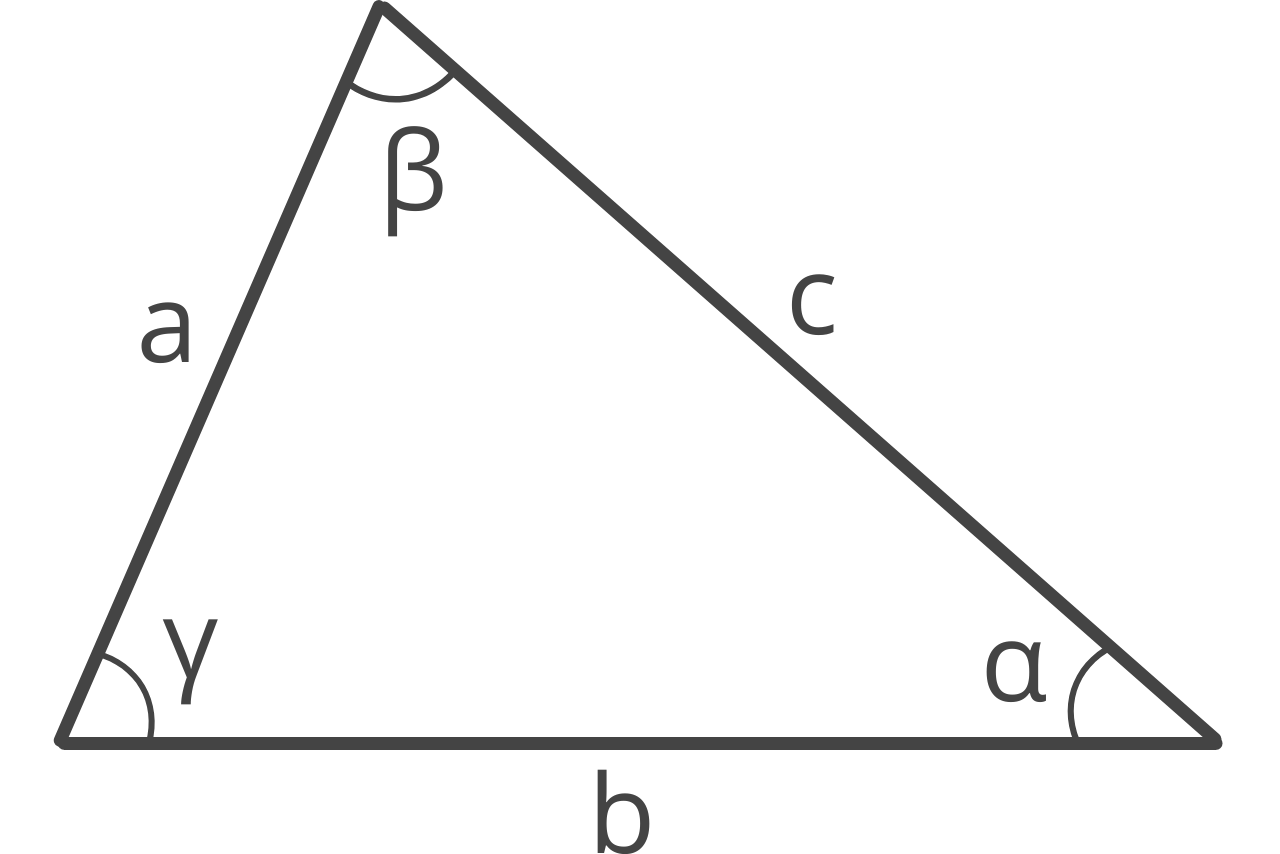# Sine Calculator – Calculate sin(x)

Find the sine of an angle using the sin calculator below. Start by entering the angle in degrees or radians.

## How to Find the Sine of an Angle

In a right triangle, the sine of angle α, or sin(α), is the ratio between the opposite side of an angle and the hypotenuse.Sine is one of the three primary trigonometric functions and is abbreviated sin.

You might be curious how to find the sine of an angle. Use the formula below to calculate sin.

### Sine Formula

The sine formula is:

sin(α) = opposite / hypotenuse = a / c

Thus, the sine of angle α in a right triangle is equal to the opposite side’s length divided by the hypotenuse.

To find the ratio of sine, simply enter the length of the opposite and hypotenuse and simplify.For example, let’s calculate the sine of angle α in a triangle with the length of the opposite side equal to 4 and the hypotenuse equal to 6.

sin(α) = 4 / 6
sin(α) = 2 / 3

## Sine Graph

If you graph the sine function for every possible angle, it forms a repeating up/down curve. This is known as a sine wave.The curve begins at the origin, (0, 0), because sin(0) = 0. As sine approaches π/2, the value increases to the maximum of 1. The value then decreases and returns back to the x-axis at π and continues to the minimum value of -1 at 3π/2. The function returns to the x-axis as it completes its period at 2π.

The sine function continues indefinitely and has a period of 2π. The maximum and minimum values occur in each period and are separated by a distance of one period.

Thus, the key points on a sine graph are (0, 0), (π/2, 1), (π, 0), (3π/2, −1), and (2π, 0).

The graphic below helps to visualize how the sine curve is formed for each angle.## Sine Table

The table below shows common angles and the sin value for each of them.

Table showing common angles and sine values for each.
0 0
15° π / 12 √6 – √2 / 4
30° π / 6 1 / 2
45° π / 4 √2 / 2
60° π / 3 √3 / 2
75° / 12 √6 + √2 / 4
90° π / 2 1
105° / 12 √6 + √2 / 4
120° / 3 √3 / 2
135° / 4 √2 / 2
150° / 6 1 / 2
165° 11π / 12 √6 – √2 / 4
180° π 0
195° 13π / 12 √6 – √2 / 4
210° / 6 1 / 2
225° / 4 √2 / 2
240° / 3 √3 / 2
255° 17π / 12 √6 + √2 / 4
270° / 2 -1
285° 19π / 12 √6 + √2 / 4
300° / 3 √3 / 2
315° / 4 √2 / 2
330° 11π / 6 1 / 2
345° 23π / 12 √6 – √2 / 4
360° 0

## Inverse Sine

The inverse of the sine function is the arcsin function. Thus, if you know the ratio of sine of an angle, you can use arcsin to find the measurement of the angle.

Arcsin can also be expressed as sin-1(x).

## Cosecant

Cosecant, on the other hand, is a separate trigonometric function that is the reciprocal of the sine value. The following formulas show the relationship between sine and cosecant.

sin(α) = opposite / hypotenuse = a / c
csc(α) = hypotenuse / opposite = c / a = 1 / sin(α)

Note that arcsin is not the same as cosecant.

You might also be interested in our cosine and tangent calculators.

## Law of Sines

The Law of Sine describes the relationship between the sides and angles of oblique (scalene) triangles.sin(α) / a = sin(β) / b = sin(γ) / c

You can use the Law of Sines to solve for the angle or side of any triangle. All you need is one angle and the side opposite of that angle.

### Do you use degrees or radians with sine?

You can use both degrees and radians with sine, but it is important to know which one you are using since each produces different results. Radians are more commonly used in mathematics and programming languages.

To use degrees, you must convert them to radians using the formula:

radians = (degrees × π) / 180

You can use our degrees to radians calculator to convert an angle to degrees if needed.

### Can you find sine without a calculator?

You can find approximate sine values without a calculator by using the sine ratio. Sin A is equal to the length of the leg opposite of angle A divided by the length of the hypotenuse.

### How do you convert sine to an angle?

To convert a sine value to an angle, you need to find the inverse of the sine function, called the arcsine function (sin-1). The arcsine function takes the sine value as input and returns the corresponding angle.

Calculators with built-in arcsine functions can be used to find the angle, which is typically given in radians. To convert the result to degrees, use the formula:

degrees = (radians × 180) / π

You can also use our radians to degrees calculator for the conversion.

### Does sine repeat?

The sine function is periodic with a period of 2π radians (or 360° in degrees), meaning its values repeat at regular intervals due to the unit circle’s periodic nature.